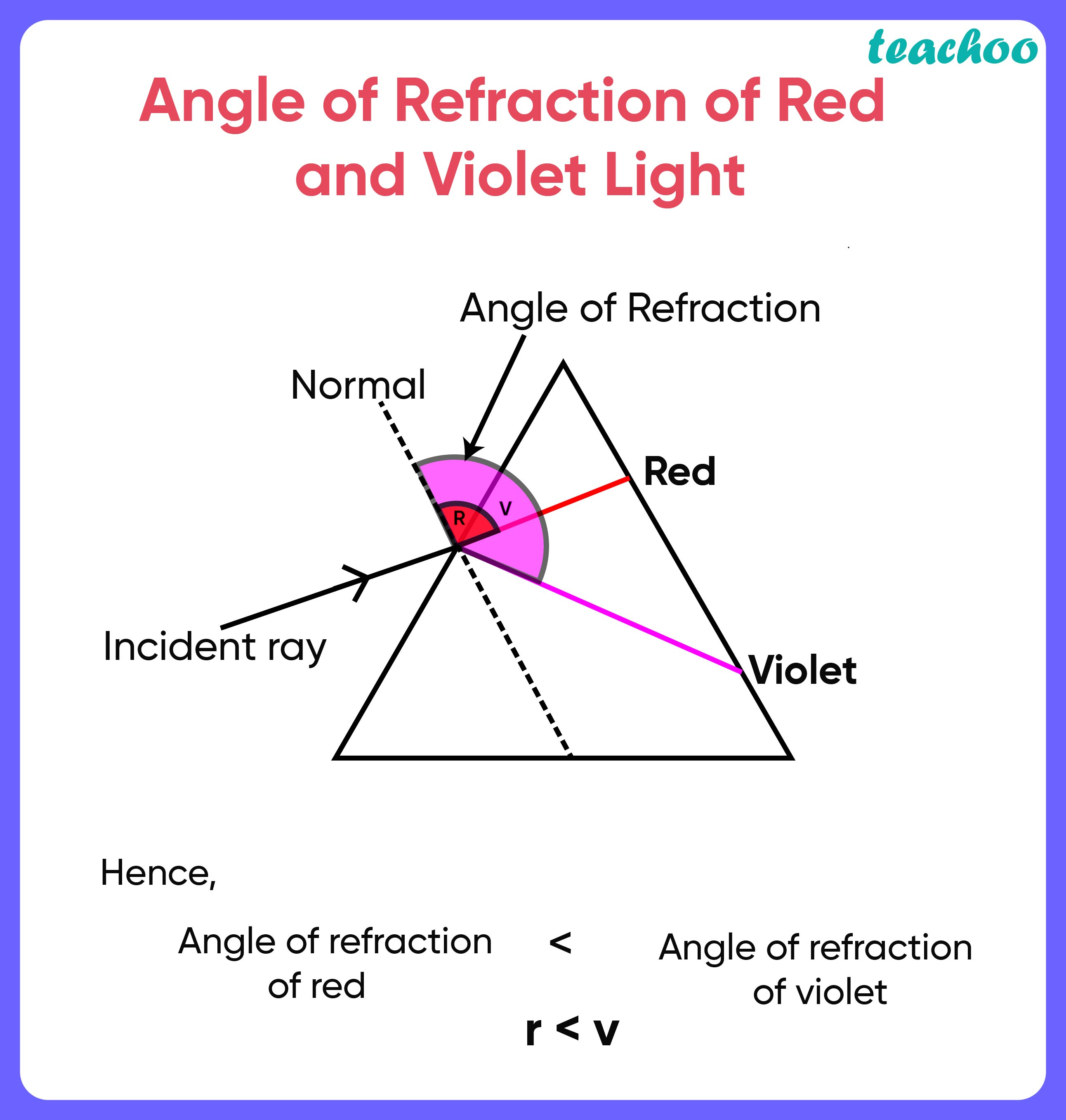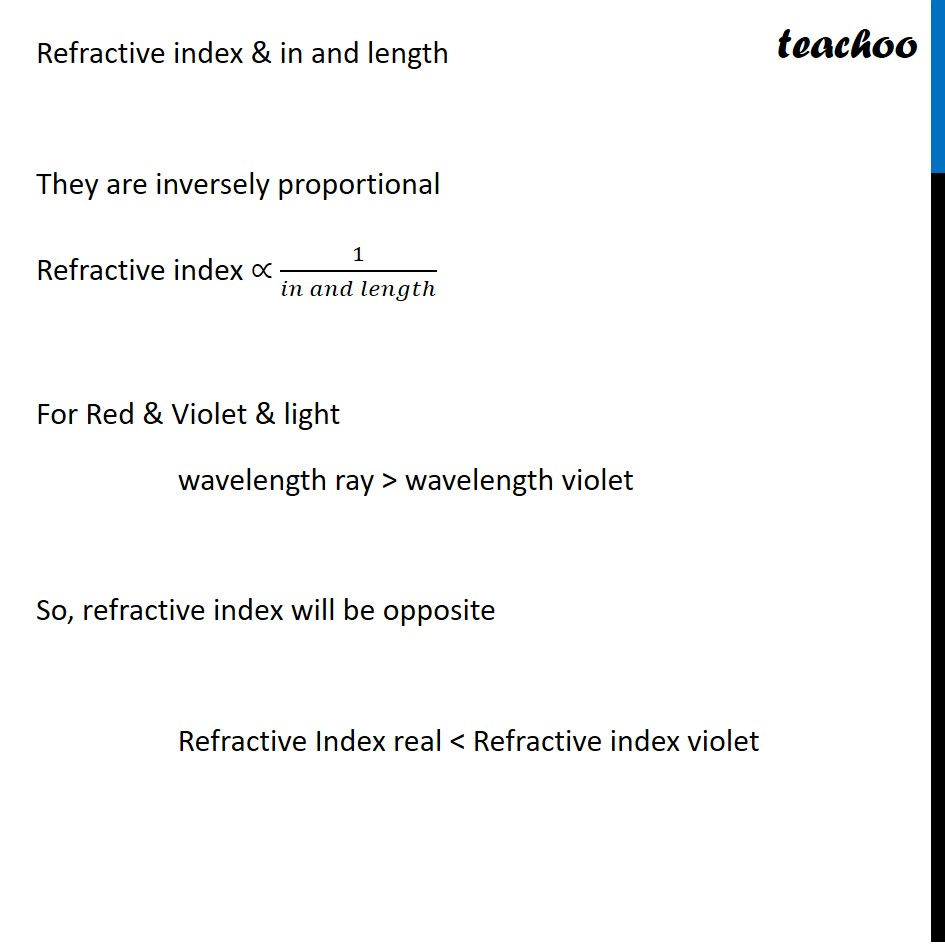Assertion Reasoning Questions (MCQ)

Class 10
Chapter 10 Class 10 - Light - Reflection and Refraction

## Reason (R): Refractive index of a pair of media depends on the wavelength of light used.

• Since angle of refraction of red and violet light is different, their Refractive index is also different• Refractive Index and Wavelength are related: Refractive index is inversely proportional to wavelengthThus,

• Assertion is true
• Reasoning is true
• And reasoning is the correct explanation of assertion

Correct option: (a)

Learn in your speed, with individual attention - Teachoo Maths 1-on-1 Class